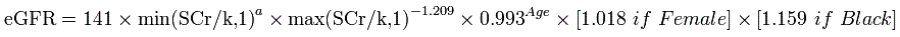Go to start of banner

# eGFR (Estimated Glomerular Filtration Rate)

Pathology > eGFR displays your data in mg/ml as a breakdown of:
Stage 1: >= 90
Stage 2: >=60 to <90
Stage 3a: >=45 to <60
Stage 3b: >=30 to <45
Stage 4: >=15 to <30
Stage 5: <15
No Data
The eGFR value is obtained through pathology results or via calculation (see below). Where both are available the latest value is displayed in the graph.
The data is displayed as a pie chart. Functions available are as described for Allergies at the beginning of this chapter.
Calculation method:
eGFR (ml/min) is calculated using the CKD-EPI formula from serum creatinine measurement (in µmol/L), age and gender. This formula iswhere SCr is serum creatinine in mg/dL (convert from µmol/L to mg/dL by dividing by 88.4)
k is 0.7 for females and 0.9 for males
a is -0.329 for females and -0.411 for males
min indicates the minimum of SCr/k or 1, and max indicates the maximum of SCr/k or 1
The CKD-EPI formula is for age >= 18.
For children the Schwartz equation is used:
GFR (mL/min/1.73 m2) = (0.41 x Height) / (Serum creatinine / 88))

# eGFR Mappings with clinical systems

The eGFR and creatinine mappings are provided in the 'General Data Mappings' Appendices under Measurements for each clinical application.